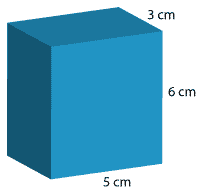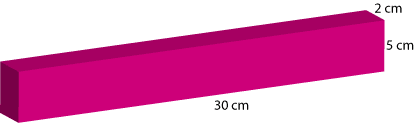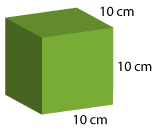#### Question 1

Calculate the volume of each rectangular prism.

1.cm3

2.cm3

3.cm3

#### Question 2

Calculate the volume of a rectangular prism with the following dimensions.

a b length = 2 cm, width = 3 cm, height = 4 cm volume = cm3 length = 3 mm, width = 5 mm, height = 2 mm volume = mm3 length = 25 m, width = 2 m, height = 4 m volume = m3

#### Question 3

Calculate the length of the missing side of each rectangular prism.

a b volume = 72m3, length = 4 m, height = 2 m What is the width? m volume = 108 cm3, length = 3 cm, height = 9 cm What is the width? cm volume = 144 m3, length = 2 m, height = 6 m What is the width? m